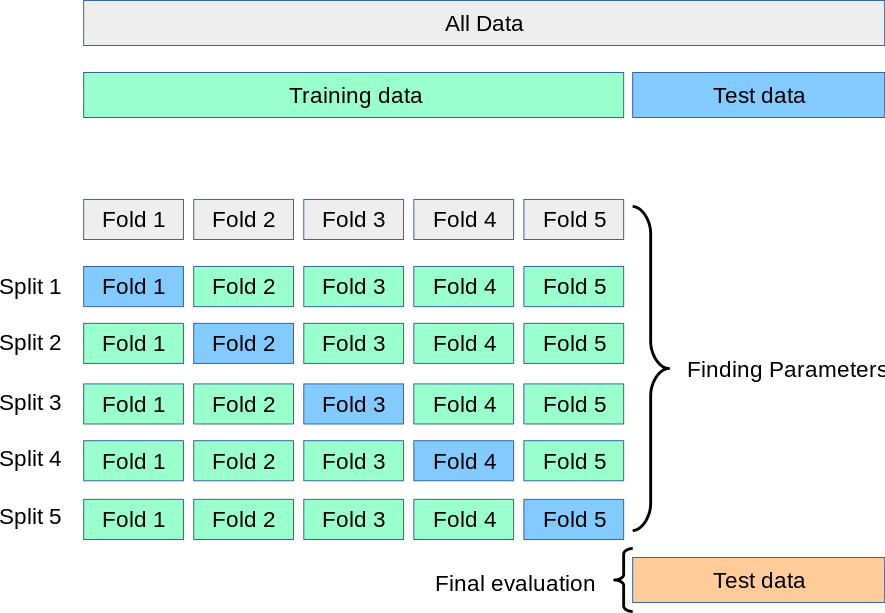# End to End ML Project - Fine tune your model with Cross Validation

In this step we will fine tune our models using cross validation. It is a resampling technique that is used to evaluate machine learning models on a limited data sample.

A test set should still be kept aside for final evaluation. We would no longer need a validation set (which is sometimes called the dev set) while doing cross validation. The training set is split into `k` smaller sets (there are other approaches too, but they generally follow the same principles). The following procedure is followed for each of the k `folds`:

• A model is trained using `k-1` of the folds as training data
• The resulting model is validated on the remaining part of the data (i.e., it is used as a test set to compute a performance measure such as accuracy)
• The performance measure reported by `k-fold cross-validation` is then the average of the values computed in the loop.

Given below is a visual representation of this process:This image is from the official page of the `Scikit-learn` cross validation where you can find more details about the process.

Now let's work on fine tuning our models using cross validation.

INSTRUCTIONS
• First, let's define a function called `display_scores` that would display the `scores`, `mean`, and `standard deviation` of all the models after applying cross validation

``````def <<your code goes here>>(scores):
print("Scores:", scores)
print("Mean:", scores.mean())
print("Standard deviation:", scores.std())
``````
• Now let's import `cross_val_score` from `Scikit-learn`

``````from sklearn.model_selection import <<your code goes here>>
``````
• Now let's calculate the cross validation scores for our `Decision Tree` model

``````scores = cross_val_score(tree_reg, housing_prepared, housing_labels,
scoring="neg_mean_squared_error", cv=10)
tree_rmse_scores = np.sqrt(-scores)
display_scores(tree_rmse_scores)
``````
• Finally, let's calculate the cross validation scores for our `Random Forest` model

``````forest_scores = cross_val_score(forest_reg, housing_prepared, housing_labels,
scoring="neg_mean_squared_error", cv=10)
forest_rmse_scores = np.sqrt(-forest_scores)
display_scores(forest_rmse_scores)
``````

No hints are availble for this assesment

Answer is not availble for this assesment

Note - Having trouble with the assessment engine? Follow the steps listed here